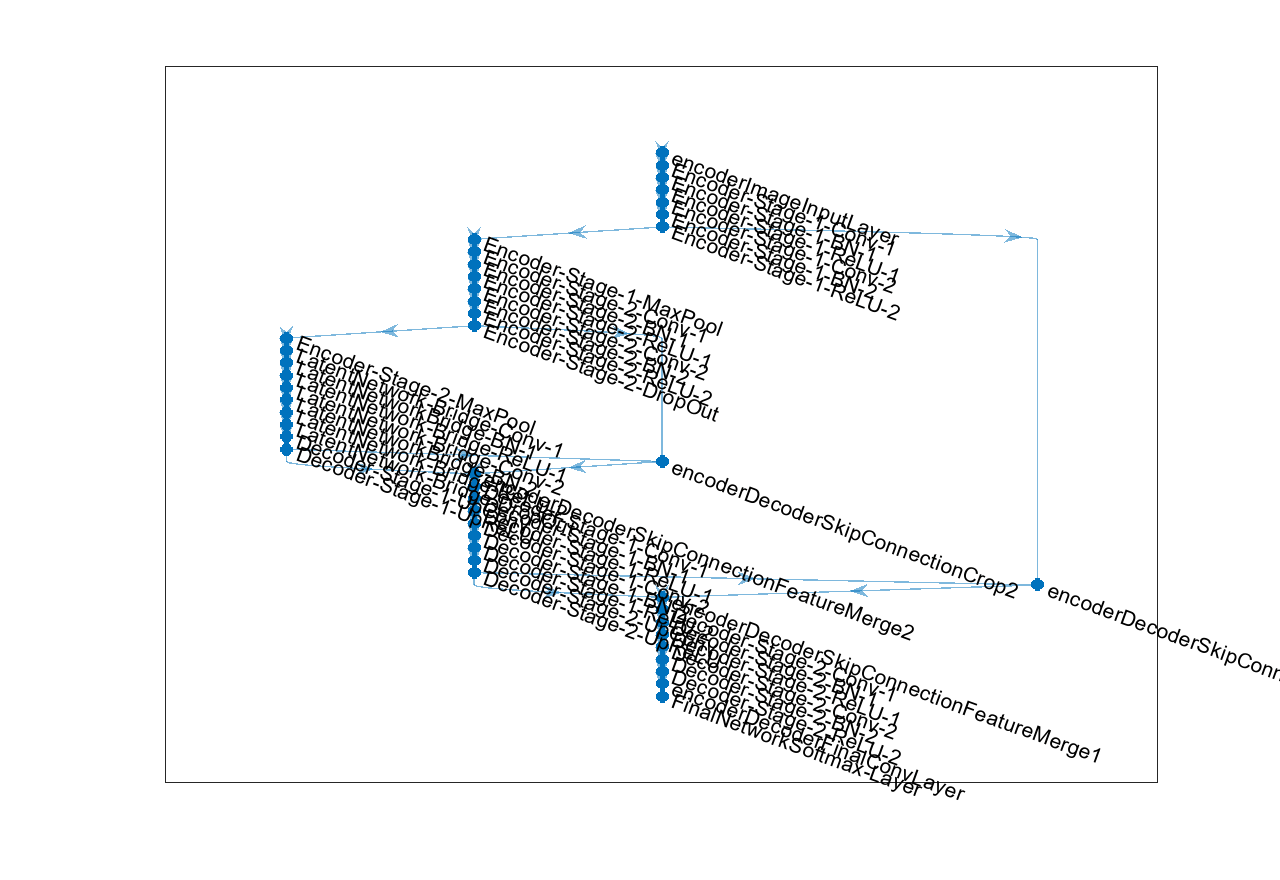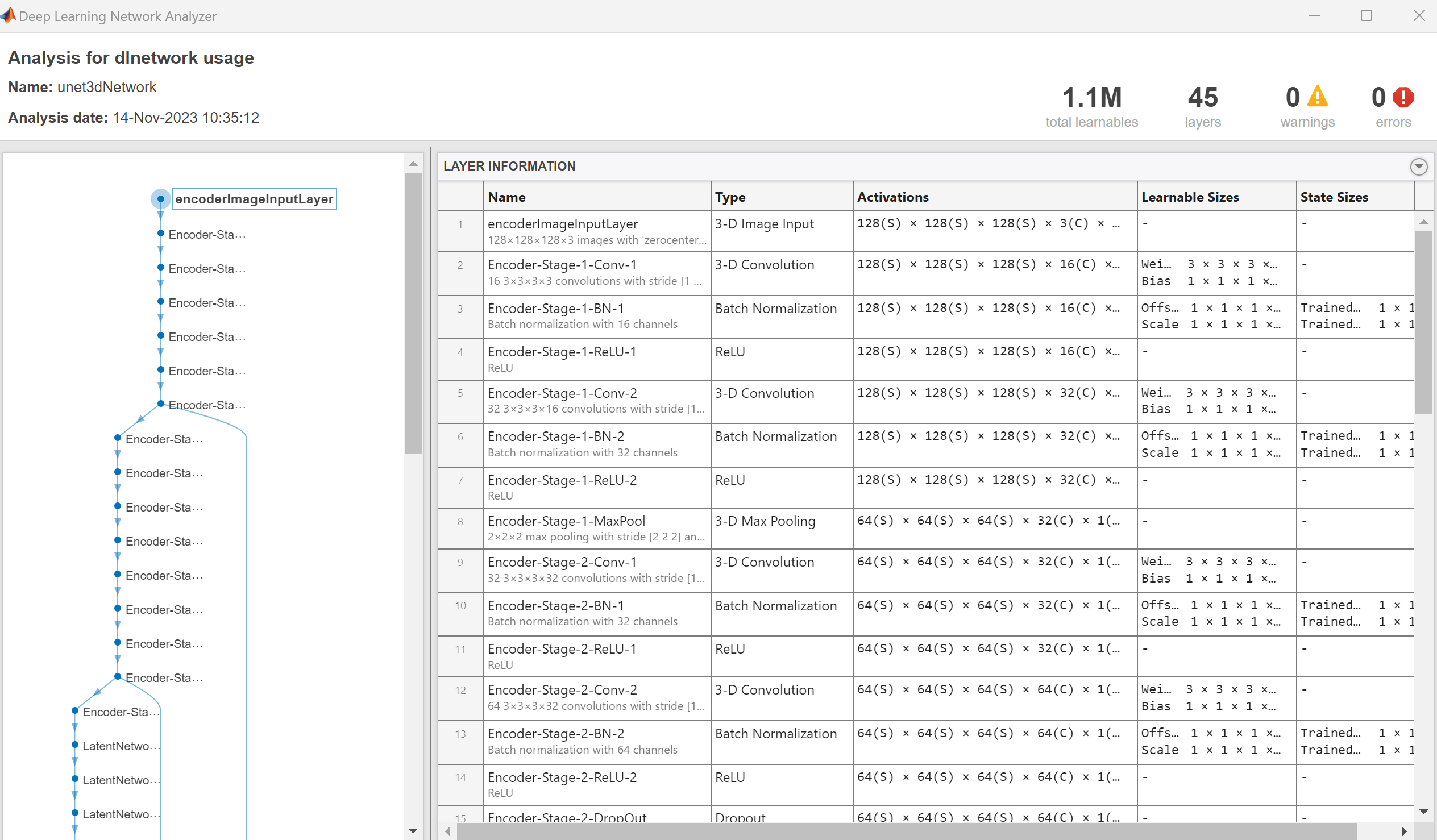# unet3dLayers

Create 3-D U-Net layers for semantic segmentation of volumetric images

## Syntax

``lgraph = unet3dLayers(inputSize,numClasses)``
``[lgraph,outputSize] = unet3dLayers(inputSize,numClasses)``
``[___] = unet3dLayers(inputSize,numClasses,Name,Value)``

## Description

````lgraph = unet3dLayers(inputSize,numClasses)` returns a 3-D U-Net network. `unet3dLayers` includes a pixel classification layer in the network to predict the categorical label for each pixel in an input volumetric image.Use `unet3dLayers` to create the network architecture for 3-D U-Net. Train the network using the Deep Learning Toolbox™ function `trainNetwork` (Deep Learning Toolbox).```

example

````[lgraph,outputSize] = unet3dLayers(inputSize,numClasses)` also returns the size of an output volumetric image from the 3-D U-Net network.```

example

````[___] = unet3dLayers(inputSize,numClasses,Name,Value)` specifies options using one or more name-value pair arguments in addition to the input arguments in previous syntax.```

## Examples

collapse all

Create a 3-D U-Net network with an encoder-decoder depth of 2. Specify the number of output channels for the first convolution layer as 16.

```imageSize = [128 128 128 3]; numClasses = 5; encoderDepth = 2; lgraph = unet3dLayers(imageSize,numClasses,'EncoderDepth',encoderDepth,'NumFirstEncoderFilters',16) ```
```lgraph = LayerGraph with properties: Layers: [40×1 nnet.cnn.layer.Layer] Connections: [41×2 table] InputNames: {'ImageInputLayer'} OutputNames: {'Segmentation-Layer'} ```

Display the network.

```figure('Units','Normalized','Position',[0 0 0.5 0.55]); plot(lgraph)```Use the deep learning network analyzer to visualize the 3-D U-Net network.

`analyzeNetwork(lgraph);`The visualization shows the number of output channels for each encoder stage. The first convolution layers in encoder stages 1 and 2 have 16 and 32 output channels, respectively. The second convolution layers in encoder stages 1 and 2 have 32 and 64 output channels, respectively.

## Input Arguments

collapse all

Network input image size representing a volumetric image, specified as one of these values:

• Three-element vector of the form [height width depth]

• Four-element vector of the form [height width depth channel]. channel denotes the number of image channels.

Note

Network input image size must be chosen such that the dimension of the inputs to the max-pooling layers must be even numbers.

Data Types: `single` | `double` | `int8` | `int16` | `int32` | `int64` | `uint8` | `uint16` | `uint32` | `uint64`

Number of classes to segment, specified as a scalar greater than 1.

Data Types: `single` | `double` | `int8` | `int16` | `int32` | `int64` | `uint8` | `uint16` | `uint32` | `uint64`

### Name-Value Arguments

Specify optional pairs of arguments as `Name1=Value1,...,NameN=ValueN`, where `Name` is the argument name and `Value` is the corresponding value. Name-value arguments must appear after other arguments, but the order of the pairs does not matter.

Before R2021a, use commas to separate each name and value, and enclose `Name` in quotes.

Example: ```unet3dLayers(inputSize,numClasses,'EncoderDepth',4) ```

Encoder depth, specified as a positive integer. The 3-D U-Net network is composed of an encoder subnetwork and a corresponding decoder subnetwork. The depth of the network determines the number of times the input volumetric image is downsampled or upsampled during processing. The encoder network downsamples the input volumetric image by a factor of 2D, where D is the value of `EncoderDepth`. The decoder network upsamples the encoder network output by a factor of 2D. The depth of the decoder subnetwork is same as that of the encoder subnetwork.

Data Types: `single` | `double` | `int8` | `int16` | `int32` | `int64` | `uint8` | `uint16` | `uint32` | `uint64`

Number of output channels for the first convolution layer in the first encoder stage, specified as a positive integer. The number of output channels for the second convolution layer and the convolution layers in the subsequent encoder stages is set based on this value.

Given stage = {1, 2, …, EncoderDepth}, the number of output channels for the first convolution layer in each encoder stage is equal to

2stage-1 `NumFirstEncoderFilters`

The number of output channels for the second convolution layer in each encoder stage is equal to

2stage `NumFirstEncoderFilters`

The `unet3dLayers` function sets the number of output channels for convolution layers in the decoder stages to match the number in the corresponding encoder stage.

Data Types: `single` | `double` | `int8` | `int16` | `int32` | `int64` | `uint8` | `uint16` | `uint32` | `uint64`

Size of the 3-D convolution filter, specified as a positive scalar integer or a three-element row vector of positive integers of the form [```fh fw fd```]. Typical values for filter dimensions are in the range [3, 7].

If you specify `'FilterSize'` as a positive scalar integer of value a, then the convolution kernel is of uniform size [```a a a```].

Data Types: `single` | `double` | `int8` | `int16` | `int32` | `int64` | `uint8` | `uint16` | `uint32` | `uint64`

Type of padding, specified as `'same'` or `'valid'`. The type of padding specifies the padding style for the `convolution3dLayer` (Deep Learning Toolbox) in the encoder and the decoder subnetworks. The spatial size of the output feature map depends on the type of padding. Specify one of these options:

• `'same'` — Zero padding is applied to the inputs to convolution layers such that the output and input feature maps are the same size.

• `'valid'` — Zero padding is not applied to the inputs to convolution layers. The convolution layer returns only values of the convolution that are computed without zero padding. The output feature map is smaller than the input feature map.

.

Note

To ensure that the height, width, and depth values of the inputs to max-pooling layers are even, choose the network input image size to confirm to any one of these criteria:

• If you specify `'ConvolutionPadding'` as `'same'`, then the height, width, and depth of the input volumetric image must be a multiple of 2D.

• If you specify `'ConvolutionPadding'` as `'valid'`, then the height, width, and depth of the input volumetric image must be chosen such that $height\text{\hspace{0.17em}}-\text{\hspace{0.17em}}\sum _{i=1}^{D}{2}^{i}\left({f}_{h}-1\right)\text{\hspace{0.17em}}$, $width\text{\hspace{0.17em}}-\text{\hspace{0.17em}}\sum _{i=1}^{D}{2}^{i}\left({f}_{w}-1\right)$, and $depth\text{\hspace{0.17em}}-\text{\hspace{0.17em}}\sum _{i=1}^{D}{2}^{i}\left({f}_{d}-1\right)$ are multiples of 2D.

where fh, fw and fd are the height, width, and depth of the three-dimensional convolution kernel, respectively. D is the encoder depth.

Data Types: `char` | `string`

## Output Arguments

collapse all

Layers that represent the 3-D U-Net network architecture, returned as a `layerGraph` (Deep Learning Toolbox) object.

Network output image size, returned as a four-element vector of the form [height, width, depth, channels]. channels is the number of output channels and is equal to the number of classes specified at the input. The height, width, and depth of the output image from the network depend on the type of padding convolution.

• If you specify `'ConvolutionPadding'` as `'same'`, then the height, width, and depth of the network output image are the same as that of the network input image.

• If you specify `'ConvolutionPadding'` as `'valid'`, then the height, width, and depth of the network output image are less than that of the network input image.

Data Types: `double`

collapse all

### 3-D U-Net Architecture

• The 3-D U-Net architecture consists of an encoder subnetwork and decoder subnetwork that are connected by a bridge section.

• The encoder and decoder subnetworks in the 3-D U-Net architecture consist of multiple stages. `EncoderDepth`, which specifies the depth of the encoder and decoder subnetworks, sets the number of stages.

• Each encoder stage in the 3-D U-Net network consists of two sets of convolutional, batch normalization, and ReLU layers. The ReLU layer is followed by a 2-by-2-by-2 max pooling layer. Likewise, each decoder stage consists of a transposed convolution layer for upsampling, followed by two sets of convolutional, batch normalization, and ReLU layers.

• The bridge section consists of two sets of convolution, batch normalization, and ReLU layers.

• The bias term of all convolution layers is initialized to zero.

• Convolution layer weights in the encoder and decoder subnetworks are initialized using the `'He'` weight initialization method.

## Tips

• Use `'same'` padding in convolution layers to maintain the same data size from input to output and enable the use of a broad set of input image sizes.

• Use patch-based approaches for seamless segmentation of large images. You can extract image patches by using the `randomPatchExtractionDatastore` function in Image Processing Toolbox™.

• Use `'valid'` padding in convolution layers to prevent border artifacts while you use patch-based approaches for segmentation.

 Çiçek, Ö., A. Abdulkadir, S. S. Lienkamp, T. Brox, and O. Ronneberger. "3D U-Net: Learning Dense Volumetric Segmentation from Sparse Annotation." Medical Image Computing and Computer-Assisted Intervention – MICCAI 2016. MICCAI 2016. Lecture Notes in Computer Science. Vol. 9901, pp. 424–432. Springer, Cham.